# 电感的计算公式

阻抗(ohm) = 2 * 3.14159 * F(工作频率) * 电感量(mH)，设定需用 360ohm 阻抗，因此：
电感量(mH) = 阻抗 (ohm) ÷ (2*3.14159) ÷ F (工作频率) = 360 ÷ (2*3.14159) ÷ 7.06 = 8.116mH
据此可以算出绕线圈数：
圈数 = [电感量* { ( 18*圈直径(吋)) + ( 40 * 圈长(吋))}] ÷ 圈直径 (吋)
圈数 = [8.116 * {(18*2.047) + (40*3.74)}] ÷ 2.047 = 19 圈
空心电感计算公式
作者：佚名 转贴自：本站原创 点击数：6684 文章录入： zhaizl
空心电感计算公式：L(mH)=(0.08D.D.N.N)/(3D+9W+10H)
D------线圈直径
N------线圈匝数
d-----线径
H----线圈高度
W----线圈宽度
单位分别为毫米和mH。。
空心线圈电感量计算公式:
l=(0.01*D*N*N)/(L/D+0.44)
线圈电感量 l单位: 微亨
线圈直径 D单位: cm
线圈匝数 N单位: 匝
线圈长度 L单位: cm
频率电感电容计算公式:
l=25330.3/[(f0*f0)*c]
工作频率: f0 单位:MHZ 本题f0=125KHZ=0.125
谐振电容: c 单位:PF 本题建义c=500...1000pf 可自行先决定,或由Q
值决定
谐振电感: l 单位: 微亨
线圈电感的计算公式
作者： 线圈电感的计算公式 转贴自：转载 点击数：299
1。针对环行CORE，有以下公式可利用: (IRON)
L=N2．AL L= 电感值（H)
H-DC=0.4πNI / l N= 线圈匝数(圈)
AL= 感应系数
H-DC=直流磁化力 I= 通过电流(A)
l= 磁路长度（cm)
l及AL值大小，可参照Micrometal对照表。例如: 以T50-52材，线圈5圈半，其L值为T50-52(表示OD为0.5英吋)，经查表其AL值约为33nH
L=33．(5.5)2=998.25nH≒1μH
当流过10A电流时，其L值变化可由l=3.74(查表)
H-DC=0.4πNI / l = 0.4×3.14×5.5×10 / 3.74 = 18.47 （查表后）
即可了解L值下降程度(μi%)
2。介绍一个经验公式
L=(k*μ0*μs*N2*S)/l
其中
μ0 为真空磁导率=4π*10(-7)。（10的负七次方）
μs 为线圈内部磁芯的相对磁导率，空心线圈时μs=1
N2 为线圈圈数的平方
S 线圈的截面积，单位为平方米
l 线圈的长度， 单位为米
k 系数，取决于线圈的半径（R)与长度(l)的比值。
计算出的电感量的单位为亨利（H）。

## 金昊德资讯中心

### 新闻中心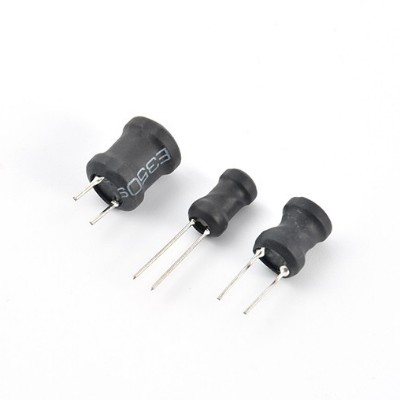#### 工字型电感-PK工字电感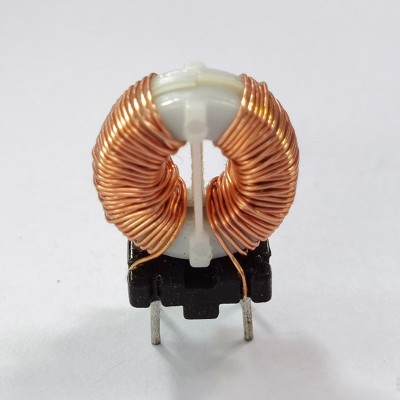#### WU非晶磁环电感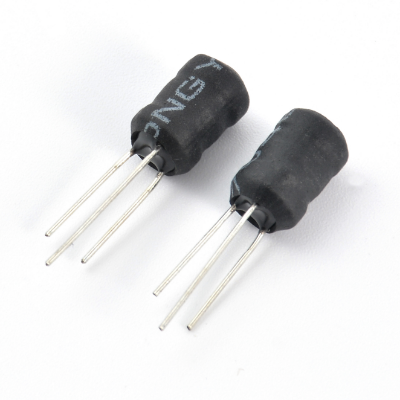#### 升压工字型电感-PK3W工字电感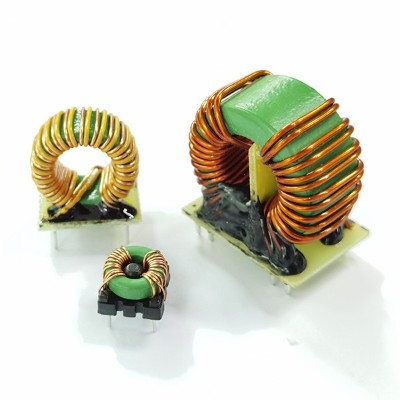#### TM锰锌磁环电感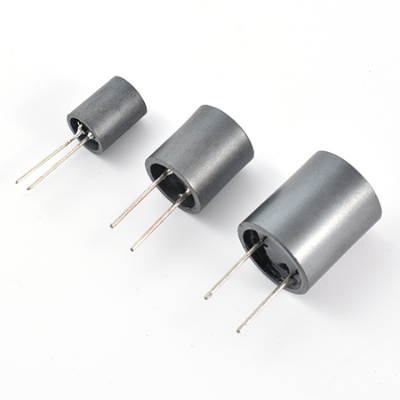#### 屏蔽工字型电感-PKF工字电感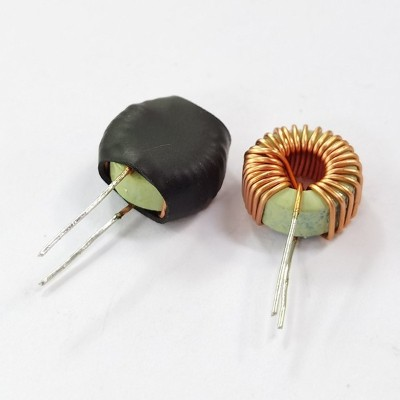#### TC铁粉芯磁环电感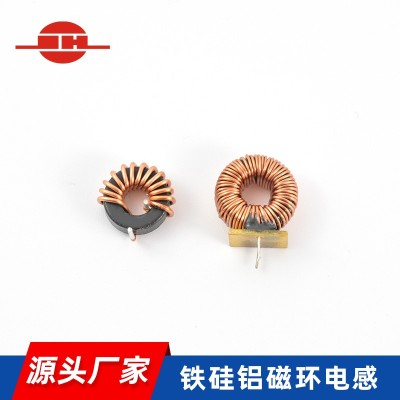#### 铁硅铝磁环电感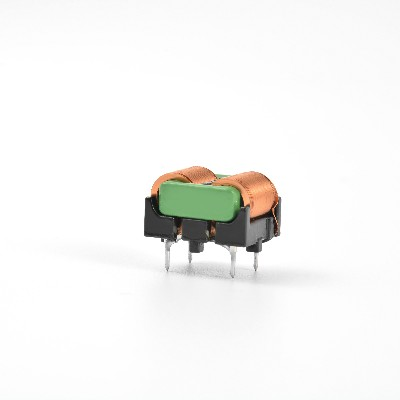#### 共模电感——SQ1212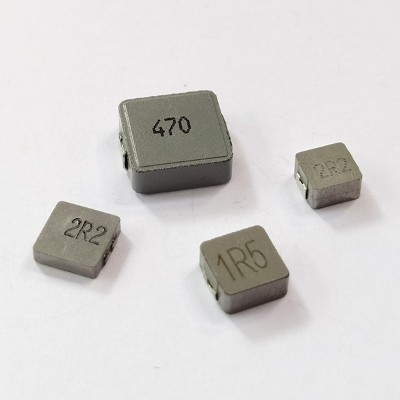#### SPL一体电感#### 共模电感——SQ1515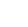全国服务热线

0755-27916126
• 咨询电话

0755-27916126